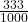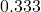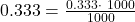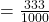## Convert the decimal to a fraction in simplest form: 0.333 O 533/1000 1 O 333 —

Question

Convert the decimal to a fraction in simplest form:
0.333

O 533/1000

1
O 333 —
10

1
O 3 —
333

O 333/1000

in progress 0
5 months 2021-08-23T10:17:20+00:00 1 Answers 9 views 0

## Answers ( )

Decimal 0.333 to a fraction in simplest form is:Step-by-step explanation:

Given the decimalMultiply and divide by 10 for every number after the decimal point.

There are three digits to the right of the decimal point, therefore multiply and divide by 1000.

Thus,∵ 0.333×1000 = 333

Let us check if we can reduce the fractionFor this, we need to find a common factor of 333 and 1000 in order to cancel it out.

But, first, we need to find the Greatest Common Divisor (GCD) of 333, 1000

Greatest Common Divisor (GCD) :

The GCD of a, b is the largest positive number that divides both a and b without a remainder.

Prime Factorization of 333:      3 · 3 · 37

Prime Factorization of 1000:      2 · 2 · 2 · 5 · 5 · 5

As there is no common factor for 333 and 1000, therefore, the GCD is 1.

Important Tip:

• As GCD is 1, therefore the fraction can not be simplified.

Therefore, decimal 0.333 to a fraction in simplest form is: